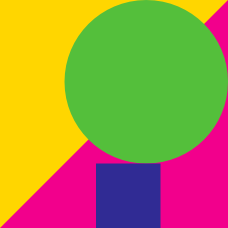Geometry

# Geometry Warmups: Level 5 Challenges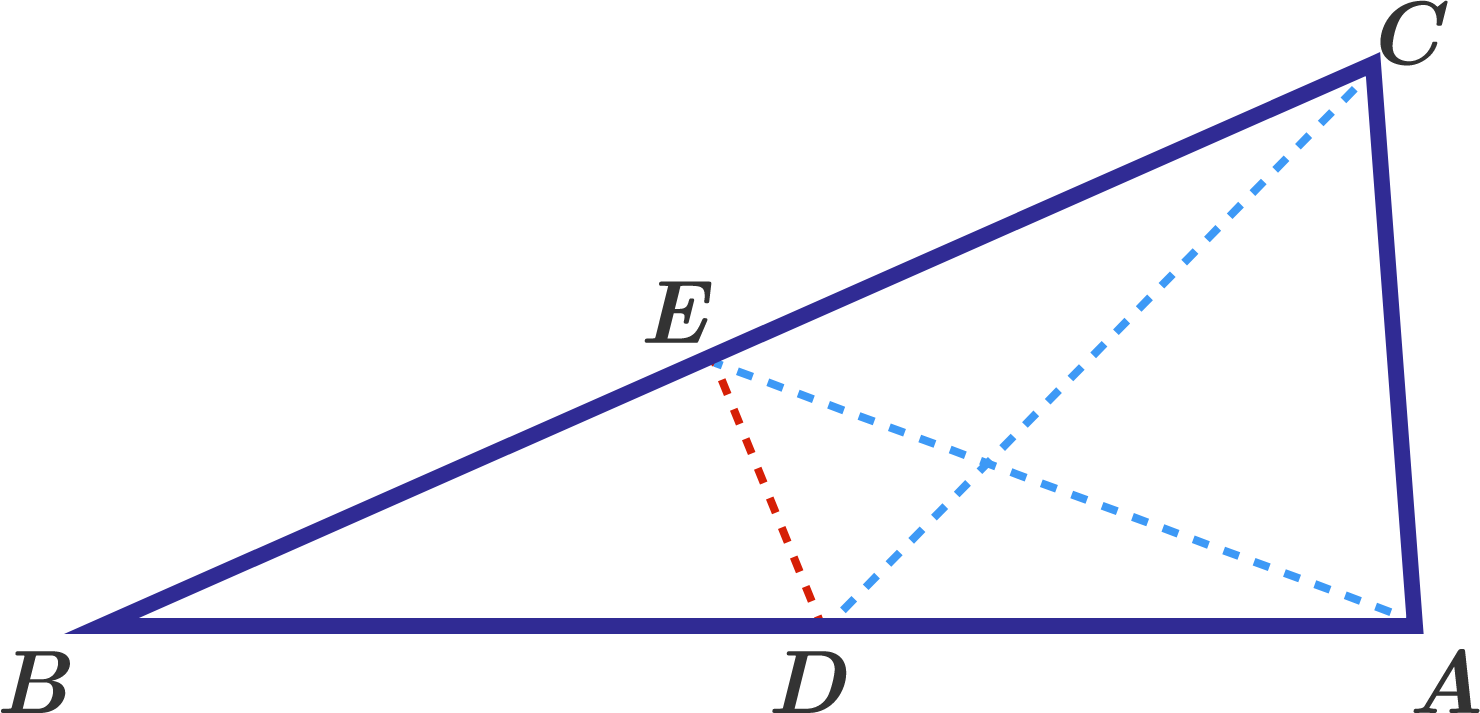In a triangle $ABC$ $\angle A =84^\circ,$ $\angle C=78^\circ.$ Points $D$ and $E$ are taken on the sides $AB$ and $BC,$ so that $\angle ACD =48^\circ,$ $\angle CAE =63^\circ.$ What is the measure (in degrees) of $\angle CDE$ ?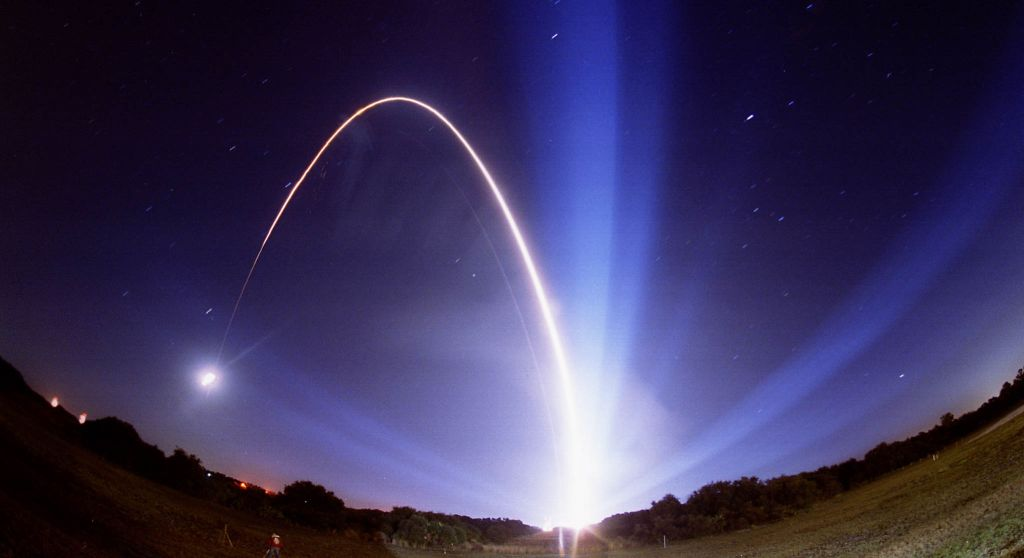A parabola passes through the following points

$\left( -1,1 \right) ,\left( 0,0 \right) ,\left( \dfrac { 1 }{ 2 } ,\dfrac { 1 }{ 4 } \right) ,\left( 1,1 \right) ,\left( -\dfrac { 7 }{ 5 } ,-\dfrac { 3 }{ 5 } \right).$

It also passes through the point: $(\dfrac { 289 }{ 240 } ,\dfrac { a }{ b } ),$ where $a,b$ are positive coprime integers. Find $a+b$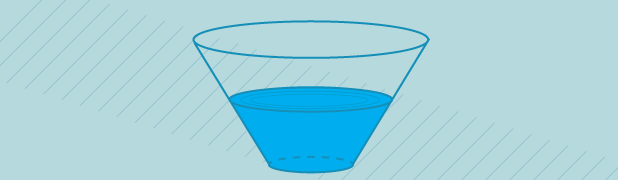Consider a glass in the shape of an inverted truncated right cone (i.e. frustrum). The radius of the base is 4, the radius of the top is 9, and the height is 7. There is enough water in the glass such that when it is tilted the water reaches from the tip of the base to the edge of the top. The proportion of the water in the cup as a ratio of the cup's volume can be expressed as the fraction $\frac{m}{n}$, for relatively prime integers $m$ and $n$. Compute $m+n$.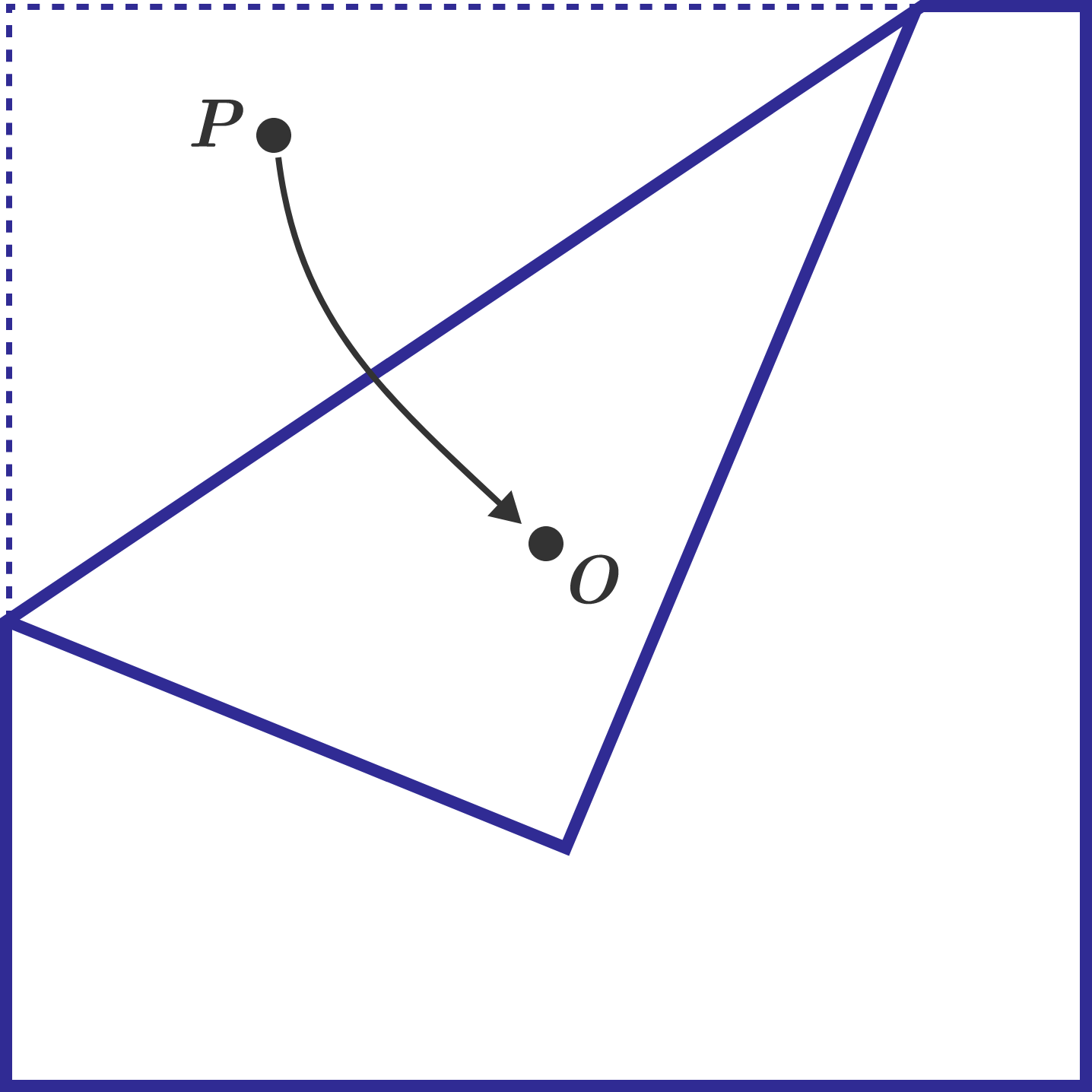A square sheet of paper has its center marked with point $O$. A random point is uniformly chosen on the square paper, and this point is labeled $P$. The paper is then folded so that point $P$ coincides with point $O$. Let the probability that a pentagon is formed after the fold be $N$. Find the value of $\lfloor 1000N \rfloor$.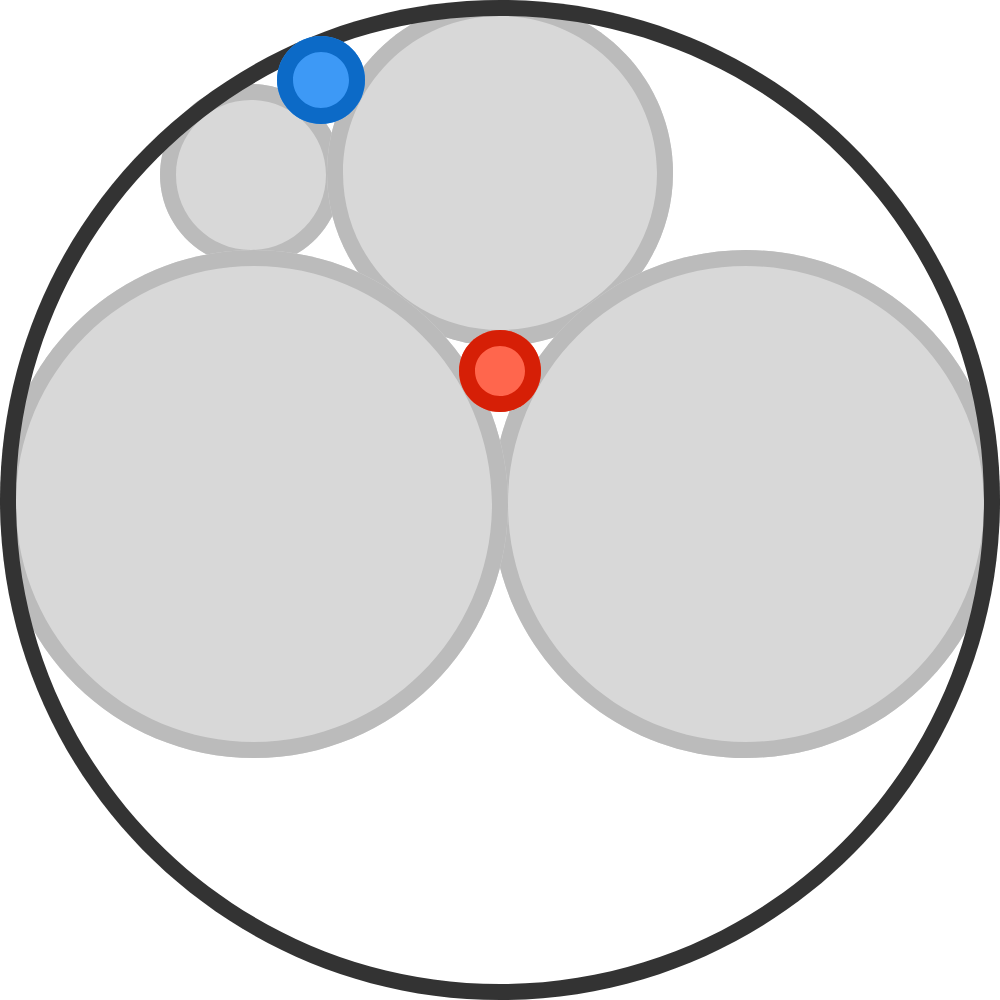The two large gray circles are congruent, and each is half the diameter of the largest circle. All circles that appear to be tangent to each other are indeed tangent to each other.

$\frac{\text{radius of blue circle}}{\text{radius of red circle}} = \frac{a}{b},$
where $a$ and $b$ are coprime positive integers. Find $a+b$.

×